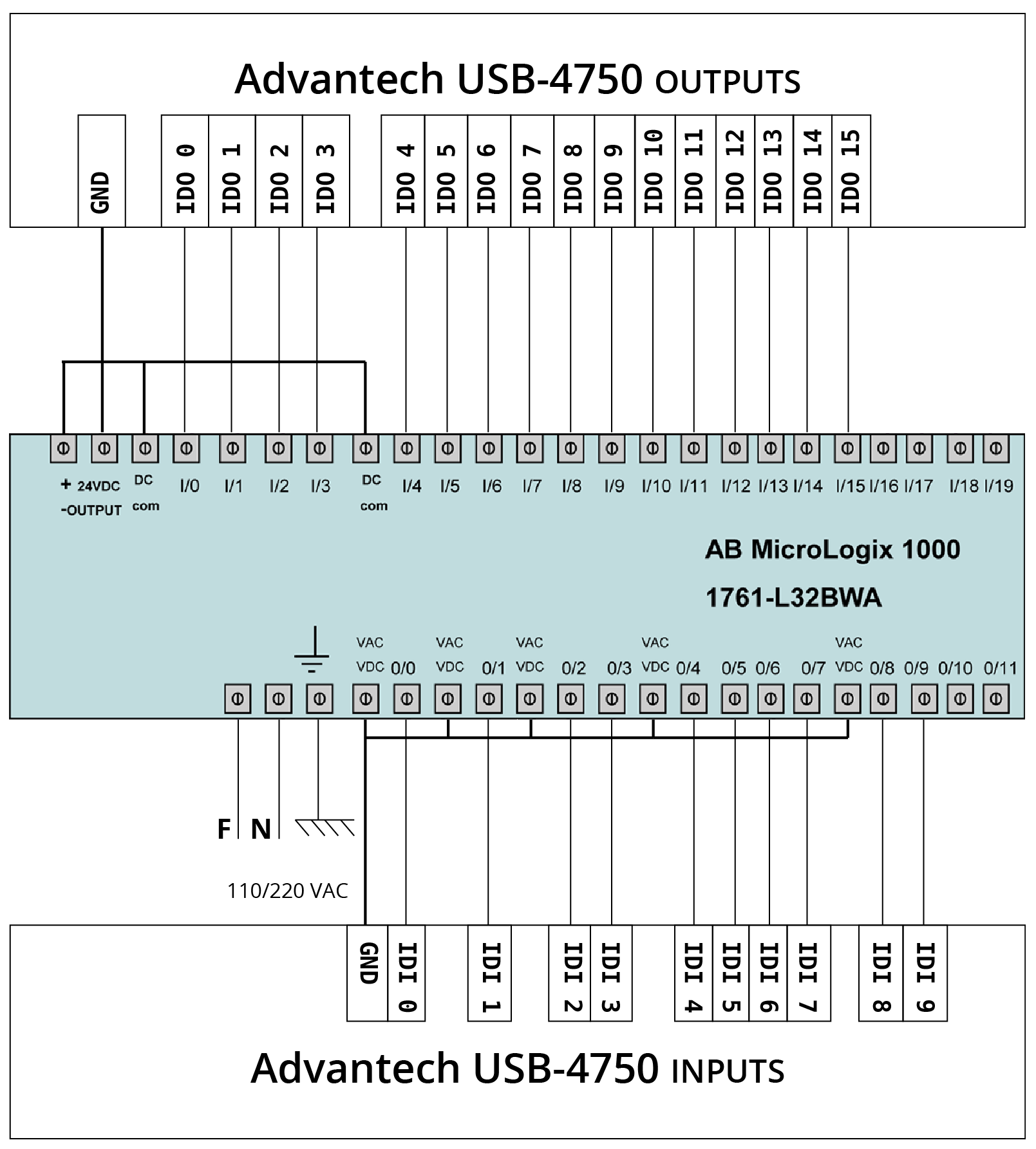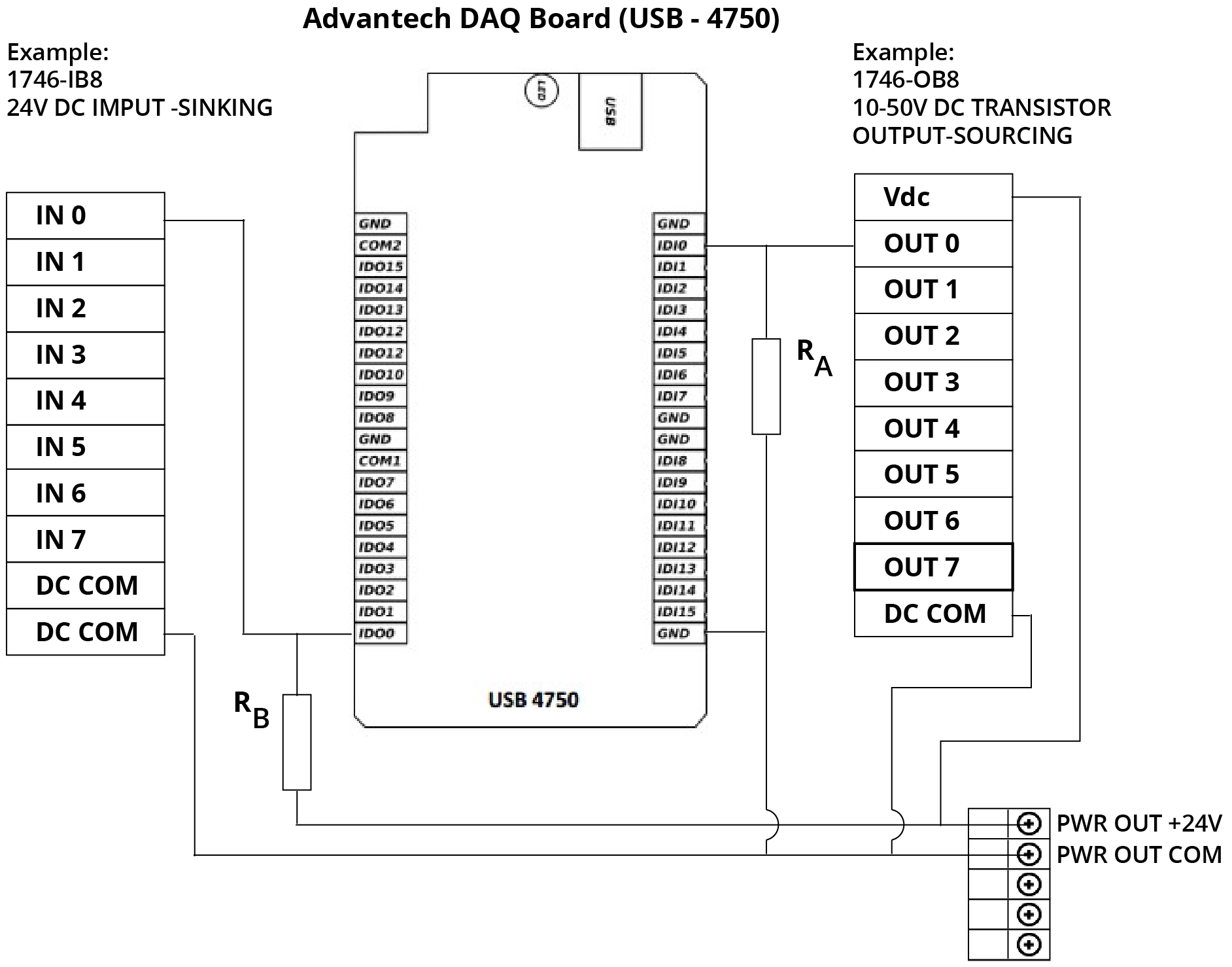## MicroLogix 10000-L32BWA¶## SLC500¶We suggest values between 1 KΩ and 4.7 KΩ with a power rating of 0.5W for the resistor values RA and RB.

The following steps show how to find the appropriate resistor's values (RA) for the PLC outputs

1. Start by using resistors with the highest possible value (4.7 KΩ)
2. Force a PLC output and check if the application detects it
3. If the application detects the forced value, you found the correct resistor, otherwise choose a lower resistor value and repeat the process
4. Repeat these steps for all outputs

The following steps show how to find the appropriate resistor's values (RB) for the PLC inputs

1. Start using resistors with the highest possible value (4.7 KΩ)
2. Force a sensor value on the application and check if PLC input detects it
3. If the PLC input detects the forced value, you found the correct resistor, otherwise choose a lower resistor value and repeat the process
4. Repeat these steps for all inputs